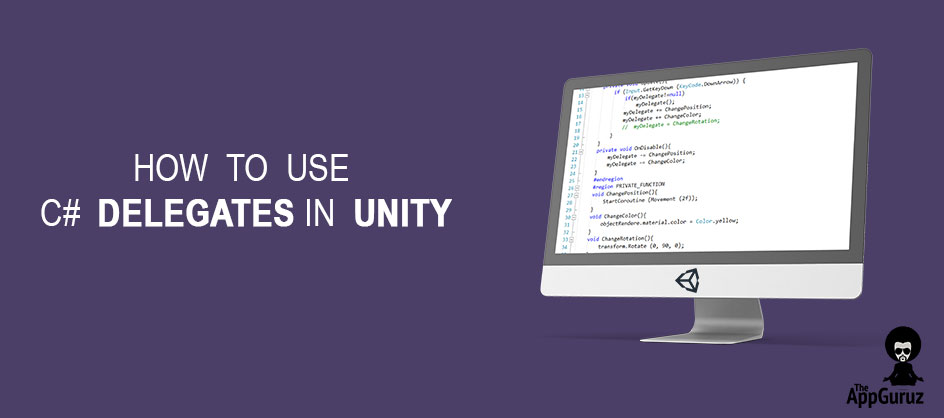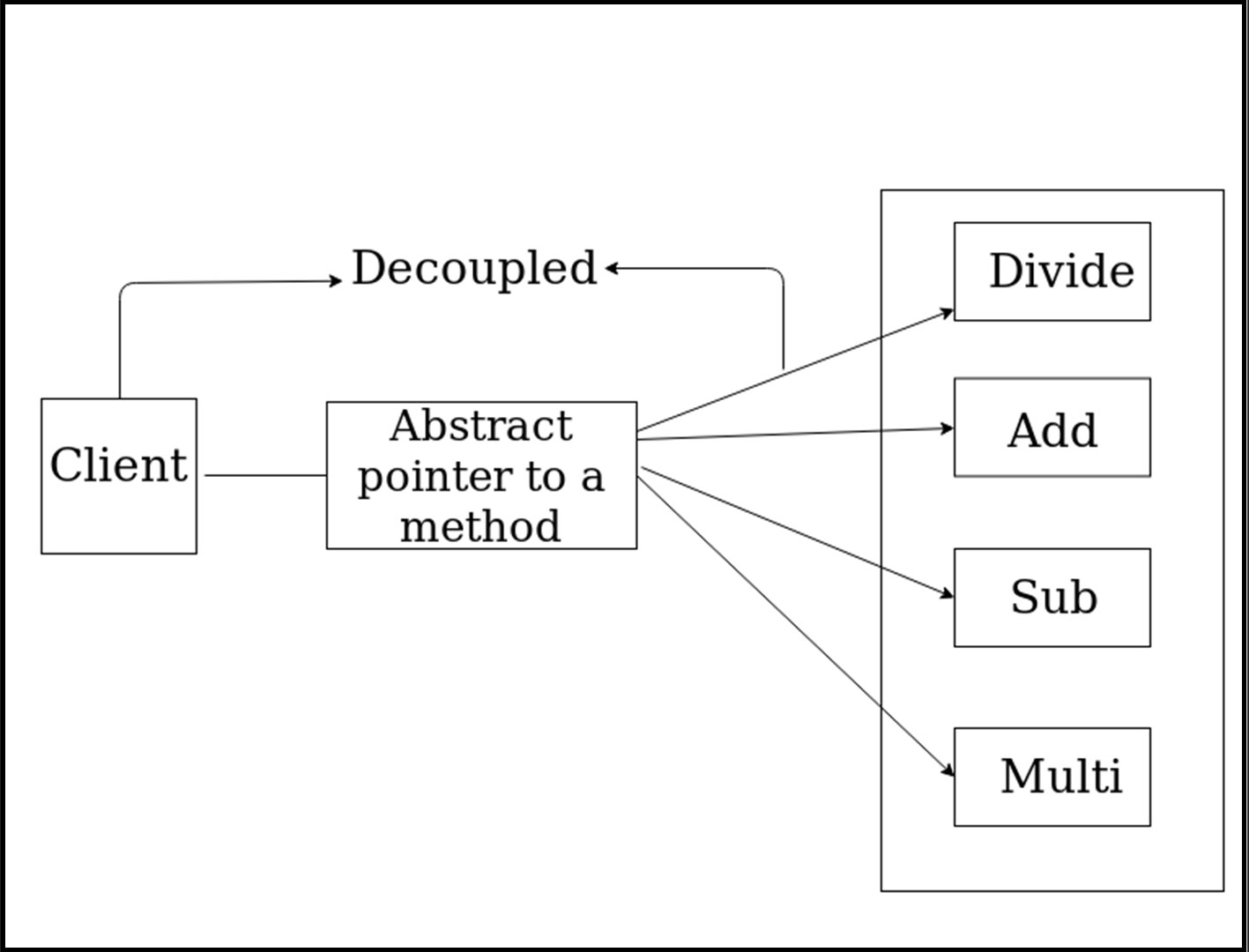## Objective

The main objective of this blog post is to give you an idea about how to use C# Delegates in Unity.

What is the problem of using functions?

What is Delegates?

Why Delegates are used?

How to declare a Delegate?

MultiCast Delegates

Problem With Multicast Delegate

Lets, try to understand that what is problem of using normal functions ?

For example,

We have one SampleDemo class which has one method called Add, which simply add two numbers.

``````public class SampleDemo : MonoBehaviour {

#region UNITY_CALLBACKS
void Update () {
if (Input.GetKeyDown (KeyCode.A)) {
}
}
#endregion

#region PUBLIC_FUNCTIONS

}
#endregion
}
``````

Let's say over period of time add Subtraction functionality to SampleDemo class ,you need to change accordingly to accommodate the new functionality.

``````public class SampleDemo : MonoBehaviour {

#region UNITY_CALLBACKS
void Update () {
if (Input.GetKeyDown (KeyCode.A)) {
}
if (Input.GetKeyDown (KeyCode.S)) {
Sub (30, 20);
}
}

#endregion

#region PUBLIC_FUNCTIONS
}
public void Sub(int num1,int num2){
Debug.Log ("Subtraction is:"+(num1-num2));
}
#endregion
}
``````

In short there is tight coupling of functions name with UI client, which want to use this functionalities.This is the main problem of using functions.

So, how we can solve this problem?

Rather than referring to actual methods, if we can refer an "abstract pointer" which turn refer to the actual methods then we can decouple the functions from UI.

This abstract pointer can be defined using Delegates.So, Now that we know it, Delegate is used to overcome the problem of tight coupling of using methods and functions.

Now, you may have Question that,

How to declare and use the delegates?

To implement a delegate is four step process, which include following steps.

1. Declare a delegate

To declare a delegate use the delegate key keyword and it must be in following manner.

``delegate void PointerToMath(int num1,int num2);``

delegate can be private or public.

the return type and input type(arguments) of delegate must be compatible,in case they are not compatible it will show the error.

2. Create a delegate reference

``PointerToMath myDelegate;``

3. Point delegate reference to the method

``myDelegate = Add;``

4. Invoke the delegate function using Invoke function of delegate

``myDelegate.Invoke (20, 30);``

You can invoke the delegate function without using Invoke function of delegate

``myDelegate(20, 30);``

Let's consider an example of delegate function which has basic calculation functionalities like Addition, Subtraction, Multiplication, Division.

Step 1

• Create a Empty GameObject and name it as you like

Step 2

• Create an empty C# script and you can name it as you like.

Write the following code in DelegatesDemo.cs.

``````public class DelegatesDemo : MonoBehaviour {

#region PRIVATE_VARIABLE
delegate void PointerToMath(int num1,int num2);
PointerToMath myDelegate;
#endregion

#region UNITY_CALLBACKS
void Update(){
if (Input.GetKeyDown (KeyCode.A)) {
myDelegate(20, 30);
}
if (Input.GetKeyDown (KeyCode.S)) {
myDelegate = Sub;
myDelegate (20, 30);
}
if (Input.GetKeyDown (KeyCode.M)) {
myDelegate = Mul;
myDelegate (20, 30);
}
if (Input.GetKeyDown (KeyCode.D)) {
myDelegate = Div;
myDelegate (20, 30);
}
}
#endregion

#region PRIVATE_FUNCTIONS
Debug.Log ("Addition of two Number is: "+(num1+num2));
}
private void Sub(int num1,int num2){
Debug.Log ("Subtraction of two Number is: "+(num1-num2));
}
private void Mul(int num1,int num2){
Debug.Log ("Multiplication of two Number is: "+(num1*num2));
}
private void Div(int num1,int num2){
Debug.Log ("Division of two Number is: "+(num1/num2));
}
#endregion
}``````

Step 3

• Assign it to the empty gameObject and run the Unity see the Console.

By pressing keys as per the code you will get the answer accordingly.

Now, you may think that here only one method call at a time what if I want to call sequence of functions with one delegate?

Here is the Solution, Multicast Delegate!!!!!!.

Multicast Delegates

Creates a delegate which can point to multiple functions and methods.

If we invoke such delegate it will invoke all the methods and functions associated with the same one after another sequentially.

Below is the code snippet which attaches 2 methods i.e. Add and Sub with delegate myDelegate.

In order to add multiple methods and function we need to use ‘+=’ symbols.

• myDelegate += Sub;

Now if we invoke the delegate it will invoke Add first and then Sub. Invocation happen in the same sequence as the attachment is done.

Let's consider a previous example of delegate function which has basic calculation functionalities like Addition, Subtraction, Multiplication, Division.

Step 1

• Create a Empty GameObject and name it as you like

Step 2

• Create an empty C# script and name it as you like.

Write the following code in MulticastDelegates.cs.

``````public class MulticastDelegates : MonoBehaviour {

#region PRIVATE_VARIABLE
delegate void PointerToMath(int num1,int num2);
PointerToMath myDelegate;
#endregion

#region UNITY_CALLBACKS
private void OnEnable(){
myDelegate += Sub;
myDelegate += Mul;
myDelegate += Div;
}
private void OnDisable(){
myDelegate -= Sub;
myDelegate -= Mul;
myDelegate -= Div;
}
void Update(){
if (Input.GetKeyDown (KeyCode.Space)) {
myDelegate (20, 30);
}
}
#endregion

#region PRIVATE_FUNCTIONS
Debug.Log ("Addition of two Number is: "+(num1+num2));
}
private void Sub(int num1,int num2){
Debug.Log ("Subtraction of two Number is: "+(num1-num2));
}
private void Mul(int num1,int num2){
Debug.Log ("Multiplication of two Number is: "+(num1*num2));
}
private void Div(int num1,int num2){
Debug.Log ("Division of two Number is: "+(num1/num2));
}
#endregion
}``````

Step 3

• Assign it to Empty Object and Execute the code.

Let's, understand the practical usage of Multicast delegate

Consider an Example Which simply change position, and Color of object

Step 1

• Create a 3D object (Cube) name it as you like.

Step 2

• Create a C# script and name it as you like.

Write the following code in ObjectCOntroller.cs.

``````public class ObjectController : MonoBehaviour {

#region PUBLIC_VARIABLES
public	Renderer objectRendere;
public delegate void  MyDelegate();
MyDelegate myDelegate;
#endregion

#region UNITY_CALLBACKS
private void OnEnable(){
myDelegate += ChangePosition;
myDelegate += ChangeColor;

}
private void Update(){
if (Input.GetKeyDown (KeyCode.DownArrow)) {
if(myDelegate!=null)
myDelegate();
}
}
private void OnDisable(){
myDelegate -= ChangePosition;
myDelegate -= ChangeColor;
}
#endregion

#region PRIVATE_FUNCTION
void ChangePosition(){
StartCoroutine (Movement (2f));
}
void ChangeColor(){
objectRendere.material.color = Color.yellow;
}
#endregion

#region CO_ROUTINE
IEnumerator Movement(float time){
float i = 0;
float rate = 1 / time;
while(i<1){
i += Time.deltaTime * rate;
transform.position = Vector3.Lerp (Vector3.zero,Vector3.up*4,i);
yield return null;
}
}
#endregion
}``````

Step 3

• Assign it to the 3D object(cube) and Execute.

In this manner, any class can subscribe to MyDelegate and get a callback, when this gets called.

Problem With Multicast Delegate

Do you try single cast Delegate after all options of Multicast Delegate?

Lets try it.

As continue with above example, only add one line after all multicast delegate, a singlecast delegate.

As shown in below code.

``````public class ObjectController : MonoBehaviour {

#region PUBLIC_VARIABLES
public	Renderer objectRendere;
public delegate void  MyDelegate();
MyDelegate myDelegate;
#endregion

#region UNITY_CALLBACKS
private void OnEnable(){
myDelegate += ChangePosition;
myDelegate += ChangeColor;
myDelegate = ChangeRotation;

}
private void Update(){
if (Input.GetKeyDown (KeyCode.DownArrow)) {
if(myDelegate!=null)
myDelegate();
}
}
private void OnDisable(){
myDelegate -= ChangePosition;
myDelegate -= ChangeColor;
}
#endregion

#region PRIVATE_FUNCTION
void ChangePosition(){
StartCoroutine (Movement (2f));
}
void ChangeColor(){
objectRendere.material.color = Color.yellow;
}
void ChangeRotation(){
transform.Rotate (0, 90, 0);
}
#endregion

#region CO_ROUTINE
IEnumerator Movement(float time){
float i = 0;
float rate = 1 / time;
while(i<1){
i += Time.deltaTime * rate;
transform.position = Vector3.Lerp (Vector3.zero,Vector3.up*4,i);
yield return null;
}
}
#endregion
}``````

Run unity, you will notice now ChangePosition and ChangeColor won't get.

Called only ChangeRotation code will run, this is because, assign operator will reset the existing invocation list, this situation is very difficult to track if multiple classes subscribe to same delegate. We can avoid this situation by using Event. Refere how to use C# events in unity.